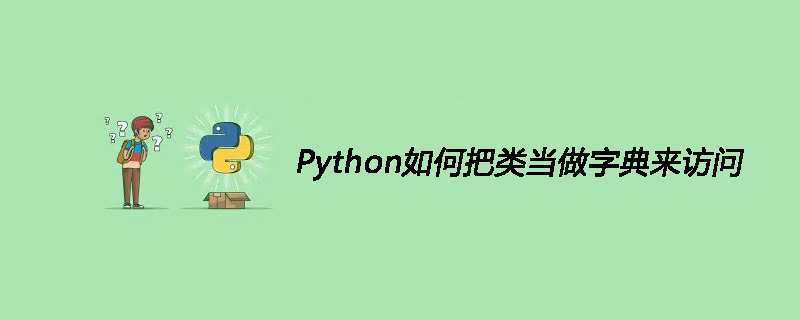# Python如何把类当做字典来访问Python把类当做字典来访问

<pre class="brush:php;toolbar:false">class A:     def __init__(self):         self.a = 1         self.b = 2 a = A() print(a.__dict__) # {'a': 1, 'b': 2} #获取变量a： print(a.__dict__['a']) # 1</pre>

<pre class="brush:php;toolbar:false">class A:     def __init__(self):         self.a = 1         self.b = 2     def keys(self):         return ('a','b',)     def __getitem__(self, item):         return getattr(self, item) a = A() print(dict(a)) #输出：{'a': 1, 'b': 2}</pre>

[]
~ ~